# 1740 I Think You’re Going to L♥ve This Multiplication Puzzle

### Today’s Puzzle:

Here’s a heart-shaped level 2 puzzle for you to do. Just place all the numbers from 1 to 10 in both the first column and the top row so that those numbers are the factors of the given clues.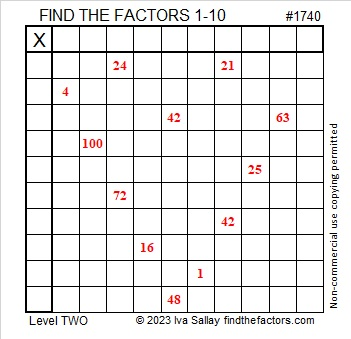Here’s the same puzzle in black and white:### Factors of 1740:

• 1740 is a composite number.
• Prime factorization: 1740 = 2 × 2 × 3 × 5 × 29, which can be written 1740 = 2² × 3 × 5 × 29.
• 1740 has at least one exponent greater than 1 in its prime factorization so √1740 can be simplified. Taking the factor pair from the factor pair table below with the largest square number factor, we get √1740 = (√4)(√435) = 2√435.
• The exponents in the prime factorization are 2, 1, 1, and 1. Adding one to each exponent and multiplying we get (2 + 1)(1 + 1)(1 + 1)(1 + 1) = 3 × 2 × 2 × 2 = 24. Therefore 1740 has exactly 24 factors.
• The factors of 1740 are outlined with their factor pair partners in the graphic below.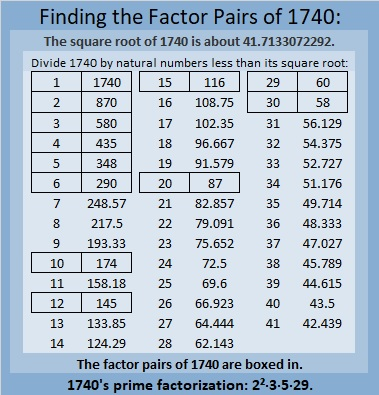### More About the Number 1740:

1740 is the hypotenuse of FOUR Pythagorean triples:
204-1728-1740, which is 3 times (68-576-580),
288-1716-1740, which is 3 times (96-572-580),
1044-1392-1740, which is (3-4-5) times 348, and
1200-1260-1740, which is (20-21-29) times 60.

1740 is palindrome 606 in base 17 because
6(17²) + 0(17) + 6(1) = 1740.

1740 is the difference of two squares in four different ways:
436² – 434² = 1740,
148² – 142² = 1740,
92² – 82² = 1740, and
44² – 14² = 1740.

# 1739 A Gift for a Valentine

### Today’s Puzzle:

Here’s a level 1 Valentine’s gift puzzle to start off the month of February. Place the numbers 1 to 10 in both the first column and the top row so that those numbers are the factors of the given number clues.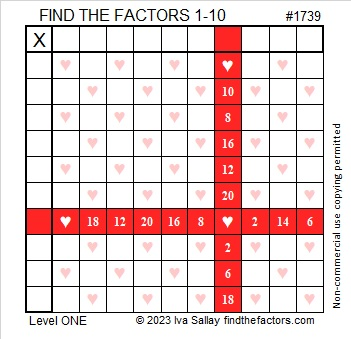And here’s a heartless copy of the same puzzle that may be more printer-friendly.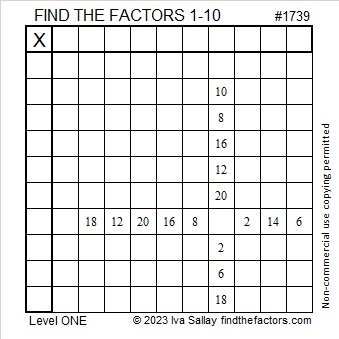### Factors of 1739:

Is 1739 a prime number? It isn’t divisible by 2 or 5.
1 + 7 + 3 + 9 = 20, so it isn’t divisible by 3.
1 – 7 + 3 – 9 = -12, so it isn’t divisible by 11.
√1739 is a little more than 41. Should I try dividing 1739 by every other prime number less than 41? I don’t know divisibility tricks for most of those prime numbers!

Here’s a shortcut I first wrote about in 341 is the smallest composite number that gives a false positive for this Quick Prime Number Test: First I type into my computer’s scientific calculator:
2  xʸ  1739  Mod  1739  =

If the answer on the calculator is anything other than “2”, then it can’t be a prime number. If it is “2”, it very likely is prime. As you can see by the screenshot of my calculator, 1739 is not prime!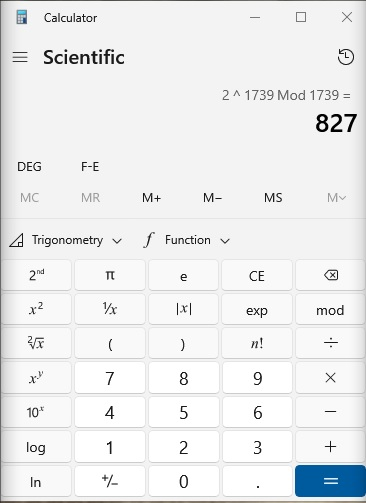• 1739 is a composite number.
• Prime factorization: 1739 = 37 × 47.
• 1739 has no exponents greater than 1 in its prime factorization, so √1739 cannot be simplified.
• The exponents in the prime factorization are 1 and 1. Adding one to each exponent and multiplying we get (1 + 1)(1 + 1) = 2 × 2 = 4. Therefore 1739 has exactly 4 factors.
• The factors of 1739 are outlined with their factor pair partners in the graphic below.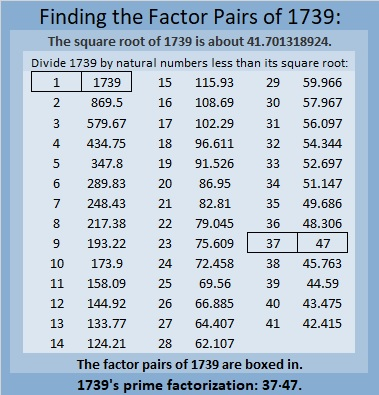### More About the number 1739:

1739 is the difference of two squares in two ways:
870² – 869² = 1739, and
42² – 5² = 1739.

That means 1739 is only 25 numbers away from the next perfect square!

# What Is Special about √1362

What is special about √1362? I don’t mean to sound like a Chevy commercial, but Just Look At It! I made this gif to show off √1362: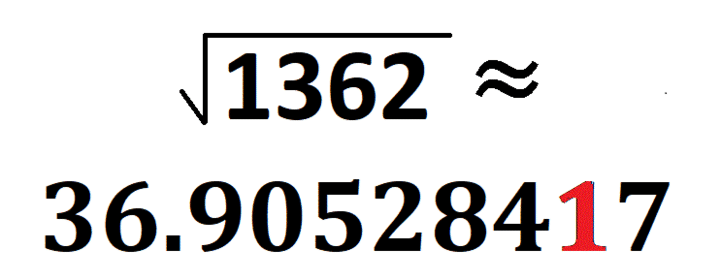make science GIFs like this at MakeaGif
1362 is the smallest number whose square root does that. Yeah, there have been some numbers that came close, but this one includes zero! Thank you OEIS.org for alerting me to that fact.
Here are a few more facts about the number 1362:
• 1362 is a composite number.
• Prime factorization: 1362 = 2 × 3 × 227
• 1362 has no exponents greater than 1 in its prime factorization, so √1362 cannot be simplified.
• The exponents in the prime factorization are 1, 1, and 1. Adding one to each exponent and multiplying we get (1 + 1)(1 + 1)(1 + 1) = 2 × 2 × 2 = 8. Therefore 1362 has exactly 8 factors.
• The factors of 1362 are outlined with their factor pair partners in the graphic below.
You can easily calculate a couple of monstrous Pythagorean triples that contain 1362:
1362-463760-463762 calculated from 2(681)(1), 681² – 1²,  681² + 1², and
1362-51520-51538 calculated from 2(227)(3), 227² – 3²,  227² + 3²

# How to Simplify √1176

### Simplifying √1176:

About 83% of numbers whose square root can be simplified are divisible by 4 or by 9 or by both. It’s easy to see rather quickly if a number is divisible by either of them. I want to find square roots by doing as few divisions as possible. It is easier to divide a number by 4 than it is to divide it by 2 twice. Likewise, it is easier to divide a number by 9 than it is to divide it by 3 twice.

1176 is divisible by 4 because the number formed from its last two digits, 76, is divisible by 4. So let’s do that division:
1176÷4=294.

Can we divide 294 by 4? We can quickly see that 94 is not divisible by 4, so 294 is not divisible by 4. Let’s check to see if it is divisible by nine: 2 + 9 + 4 = 15, which is not divisible by 9 but is by 3. That means that 294 is not divisible by 9, but it is divisible by 3.

Since 294 is an even number that is divisible by 3, I will save time and make fewer mistakes if I just divide it by 6 instead of by 2 and then by 3.
294÷6 = 49

49 is a perfect square so I know I’ve done enough dividing for this particular number. I’ve made a gif to illustrate the steps I’ve just explained:make science GIFs like this at MakeaGif

### Factor Trees for 1176:

By doing just a little bit of arithmetic in my head, I can also tell by looking at that cake that the prime factorization of 1176 is 2³ × 3 × 7².

Some people still prefer to use factor trees to find square roots. Here are a few of its MANY possible trees.### Factors of 1176:

• 1176 is a composite number.
• Prime factorization: 1176 = 2 × 2 × 2 × 3 × 7 × 7, which can be written 1176 = 2³ × 3 × 7²
• The exponents in the prime factorization are 3, 1 and 2. Adding one to each and multiplying we get (3 + 1)(1 + 1)(2 + 1) = 4 × 2 × 3 = 24. Therefore 1176 has exactly 24 factors.
• Factors of 1176: 1, 2, 3, 4, 6, 7, 8, 12, 14, 21, 24, 28, 42, 49, 56, 84, 98, 147, 168, 196, 294, 392, 588, 1176
• Factor pairs: 1176 = 1 × 1176, 2 × 588, 3 × 392, 4 × 294, 6 × 196, 7 × 168, 8 × 147, 12 × 98, 14 × 84, 21 × 56, 24 × 49 or 28 × 42
• Taking the factor pair with the largest square number factor, we get √1176 = (√196)(√6) = 14√6 ≈ 34.29286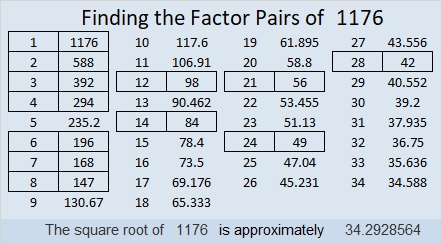### Sum-Difference Puzzles:

6 has two factor pairs. One of those pairs adds up to 5, and the other one subtracts to 5. Put the factors in the appropriate boxes in the first puzzle.

1176 has twelve factor pairs. One of the factor pairs adds up to ­70, and a different one subtracts to 70. If you can identify those factor pairs, then you can solve the second puzzle!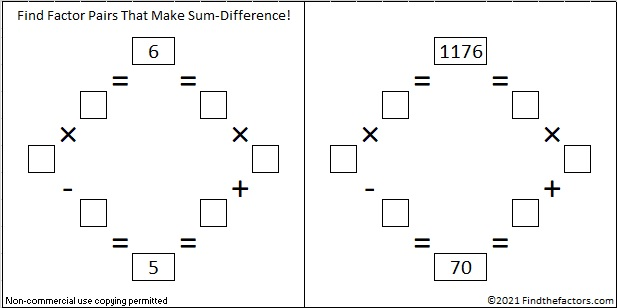The second puzzle is really just the first puzzle in disguise. Why would I say that?

### More about the Number 1176:

1176 is the sum of the first 48 numbers so we say it the 48th triangular number. We know it is the sum of the first 48 numbers because (48 × 49)/2 = 1176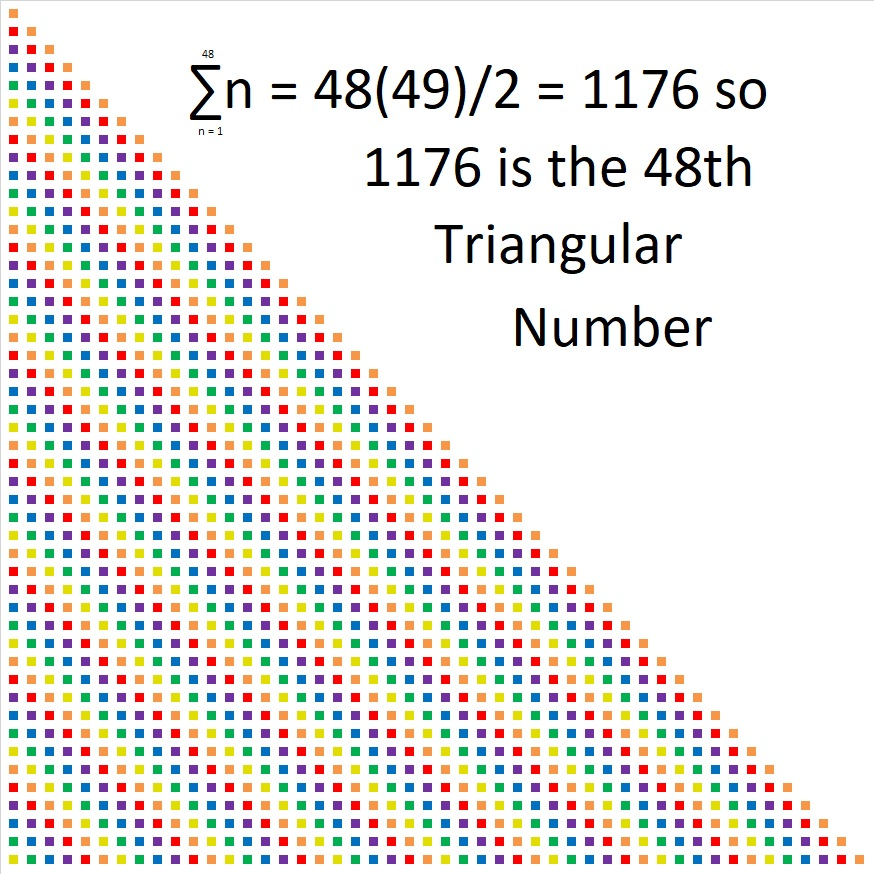1176 looks interesting in a few other bases:
It’s 3300 in BASE 7 because 3(7³ + 7²) = 3(392) = 1176,
6C6 in BASE 13 (C is 12 base 10) because 6(13²) + 12(13) + 6(1) = 1176,
600 in BASE 14 because 6(14²) = 1176,
1M1 in BASE 25 (M is 22 base 10) because 25² + 22(25) + 1 = 1176
and it looks like one of its factors, 196, in BASE 30
because 1(30²) + 9(30) + 6(1) = 1176

What’s special about √1024? Is it because it and several counting numbers after it have square roots that can be simplified?Perhaps.

Maybe it is interesting just because √1024 = 32, a whole number. The 5th root of 1024 = 4 and the 10th root of 1024 = 2, both whole numbers as well.

Those equations are true because 32² = 1024, 4⁵ = 1024, and 2¹⁰ = 1024.

Or perhaps 1024 is special because it is the smallest number that is a 10th power. (It is 2¹⁰.) The square root of a perfect 10th power is always a perfect 5th power. (32 = 2⁵ and is the smallest number that is a 5th power.)

1024 is also the smallest number with exactly 11 factors.

It is the smallest number whose factor tree has at least 10 leaves that are prime numbers. (They are the red leaves on the factor tree shown below.) It is possible to draw several other factor trees for 1024, but they will all have the number 2 appearing ten times.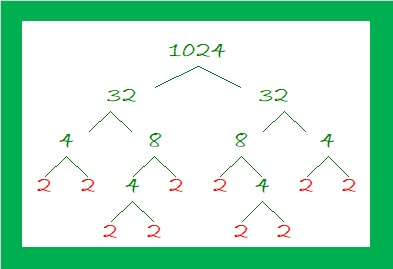What’s more, I noticed something about 1024 and some other multiples of 256: Where do multiples of 256 fall on the list of square roots that can be simplified?

• 256 × 1 = 256 and 256 is the 100th number on this list of numbers whose square roots can be simplified.• 256 × 2 = 512. When we add the next 100 square roots that can be simplified, 512 is the 199th number on the list.• Here are the third 100 square roots that can be simplified:• 256 × 3 = 768 didn’t quite make that list because it is the 301st number. Indeed, it is the first number on this list of the fourth 100 numbers whose square roots can be simplified.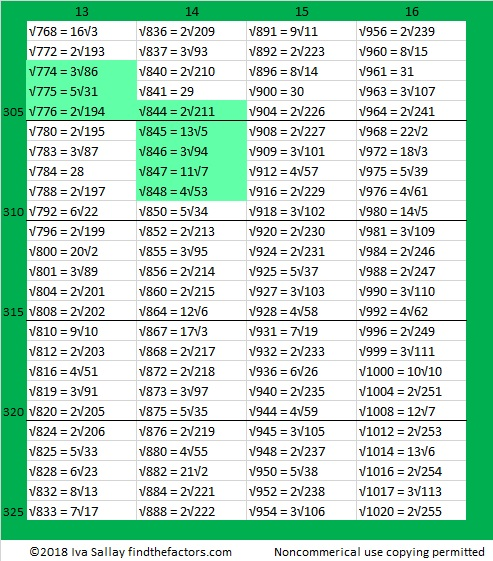• 256 × 4 = 1024. That will be the first number on the 5th 100 square roots list!

It is interesting that those multiples of 256 have the 100th, the 199th, the 301st, and the 401st positions on the list. That is so close to the 100th, 200th, 300th, and 400th positions.

In case you couldn’t figure it out, the highlighted square roots are three or more consecutive numbers that appear on the list.

1024 is interesting for many other reasons. Here are a few of them:

(4-2)¹⁰ = 1024, making 1024 the 16th Friedman number.

I like to remember that 2¹⁰ = 1024, which is just a little bit more than a thousand. Likewise 2²⁰ = 1,048,576 which is about a million. 2³⁰ is about a billion, and 2⁴⁰ is about a trillion.

*******
As stated in the comments, Paula Beardell Krieg shared a related post with me. It takes exactly 1024 Legos to build this fabulous pyramidal fractal:

1024 has so many factors that are divisible by 4 that it is a leg in NINE Pythagorean triples:
768-1024-1280 which is (3-4-5) times 256
1024-1920-2176 which is (8-15-17) times 128
1024-4032-4160 which is (16-63-65) times 64
1024-8160-8224 which is (32-255-257) times 32
1024-16368-16400 which is 16 times (64-1023-1025)
1024-32760-32776 which is 8 times (128-4095-4097)
1024-65532-65540 which is 4 times (256-16383-16385)
1024-131070-131074 which is 2 times (512-65535-65537),
and primitive 1024-262143-262145

Some of those triples can also be found because 1024 is the difference of two squares four different ways:
257² – 255² = 1024
130² – 126² = 1024
68² – 60² = 1024
40² – 24² = 1024
To find out which difference of two squares go with which triples, add the squares instead of subtracting and you’ll get the hypotenuse of the triple.
******

1024 looks interesting in some other bases:
It’s 1000000000 in BASE 2,
100000 in BASE 4,
2000 in BASE 8,
1357 in BASE 9,
484 in BASE 15,
400 in BASE 16,
169 in BASE 29,
144 in BASE 30,
121 in BASE 31, and
100 in BASE 32

• 1024 is a composite number.
• Prime factorization: 1024 = 2 × 2 × 2 × 2 × 2 × 2 × 2 × 2 × 2 × 2, which can be written 1024 = 2¹⁰
• The exponent in the prime factorization is 10. Adding one we get (10 + 1) = 11. Therefore 1024 has exactly 11 factors.
• Factors of 1024: 1, 2, 4, 8, 16, 32, 64, 128, 256, 512, 1024
• Factor pairs: 1024 = 1 × 1024, 2 × 512, 4 × 256, 8 × 128, 16 × 64, or 32 × 32,
• 1024 is a perfect square. √1024 = 32. It is also a perfect 5th power, and a perfect 10th power.# Palindrome 868 Has a Fun Square Root

Obviously, 868 is a palindrome in base 10. It has some interesting representations in some other bases, too:

• 868 is 4004 in BASE 6, because 4(6³) + 0(6²) + 0(6¹) + 4(6º) = 868
• SS in BASE 30 (S is 28 base 10), because 28(30) + 28(1) = 28(31) = 868
• S0 in BASE 31, because 28(31) = 868

From OEIS.org I learned that all the digits from 1 to 9 make up the first nine decimal places of √868, so I decided to make a gif showing that fun fact:make science GIFs like this at MakeaGif
• 868 is a composite number.
• Prime factorization: 868 = 2 × 2 × 7 × 31, which can be written 868 = 2² × 7 × 31
• The exponents in the prime factorization are 2, 1, and 1. Adding one to each and multiplying we get (2 + 1)(1 + 1)(1 + 1) = 3 × 2 × 2 = 12. Therefore 868 has exactly 12 factors.
• Factors of 868: 1, 2, 4, 7, 14, 28, 31, 62, 124, 217, 434, 868
• Factor pairs: 868 = 1 × 868, 2 × 434, 4 × 217, 7 × 124, 14 × 62, or 28 × 31,
• Taking the factor pair with the largest square number factor, we get √868 = (√4)(√217) = 2√217 ≈ 29.461839725# 671 is the Magic Sum of an 11 x 11 Magic Square

6 – 7 + 1 = 0 so 671 is divisible by 11.

671 is the sum of the fifteen prime numbers from 17 to 73.

Because 61 is one of its factors, 671 is the hypotenuse of the Pythagorean triple 121-660-671. The greatest common factor of those three numbers practically jumps out at me. Does it do the same thing to you?

Best of all 671 is the magic sum of an 11 x 11 magic square. (That link from wikipedia helped me construct this square. I’ll give directions so you can do it, too!)Notice how every row, column, and diagonal on the square sums to 671. The reason it is the magic sum is because the sum of all the numbers from 1 to 121 can be computed and then divided by 11 (the number of rows). Here is the equation:

• 671 = 121 x 120/2/11

Because 11 is an odd number there are simple directions to complete the entire square:

The number 1 is located in the exact center of the top row.

Find the number 2 on the square. (It’s located on the bottom row just right of the exact center square.) Notice that the numbers 3, 4, 5, and 6 are on the same diagonal. If you imagine the diagonal wrapping around the square, you can continue to follow it for numbers 7, 8, 9, 10, and 11. We can’t put the number 12 along the same diagonal because the number 1 is already in that spot, so we put the 12 UNDER the 11 and begin working on a new diagonal.

Anytime a number already occupies a space on a diagonal, put the next number under the preceding number and continue making a new diagonal. When a diagonal reaches the edge of the square, imagine that edge is connected to the opposite edge and continue the diagonal from the opposite edge.

I found it to be the trickiest placing the numbers 67 and 68, but other than that it was rather easy to know where to put the numbers.

Notice that the difference between any smaller number and the larger number just below it is either 12 or 1.

If you have excel on your computer, click on 12 Factors 2015-11-02, select the magic square tab, and then you can make this 11 x 11 magic square yourself. As you type in numbers, the columns, rows, and diagonals will automatically keep a running sum.

Once you get the square to give the magic sum in each direction, you can try doing the same thing with the 13 x 13 magic square that I’ve included on the same page. Its magic sum is 1105 which can be also be computed:

• 1105 = 169 x 170/22/13.

There is actually many more possible and probably more complicated 11 x 11 and 13 x 13 magic squares. I hope you enjoy making some with this easy method.

—————————————————————————————————

• 671 is a composite number.
• Prime factorization: 671 = 11 x 61
• The exponents in the prime factorization are 1 and 1. Adding one to each and multiplying we get (1 + 1)(1 + 1) = 2 x 2 = 4. Therefore 671 has exactly 4 factors.
• Factors of 671: 1, 11, 61, 671
• Factor pairs: 671 = 1 x 671 or 11 x 61
• 671 has no square factors that allow its square root to be simplified. √671 ≈ 25.903668.—————————————————————————————————

# 564 and Level 6

Consecutive primes 281 and 283 add up to 564.

564 is made from three consecutive numbers so it can be evenly divided by 3. If the middle number is divisible by 3, then a number made from three consecutive numbers will also be divisible by 9. Is 564 divisible by 9? Why or why not?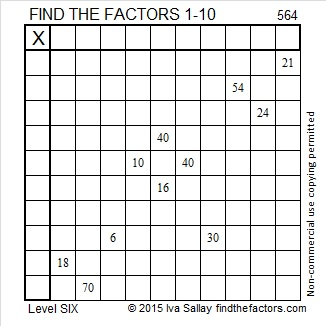Print the puzzles or type the solution on this excel file: 10 Factors 2015-07-20

—————————————————————————————————

• 564 is a composite number.
• Prime factorization: 564 = 2 x 2 x 3 x 47, which can be written 564 = (2^2) x 3 x 47
• The exponents in the prime factorization are 2, 1, and 1. Adding one to each and multiplying we get (2 + 1)(1 + 1)(1 + 1) = 3 x 2 x 2 = 12. Therefore 564 has exactly 12 factors.
• Factors of 564: 1, 2, 3, 4, 6, 12, 47, 94, 141, 188, 282, 564
• Factor pairs: 564 = 1 x 564, 2 x 282, 3 x 188, 4 x 141, 6 x 94, or 12 x 47
• Taking the factor pair with the largest square number factor, we get √564 = (√4)(√141) = 2√141 ≈ 23.74868—————————————————————————————————# 563 and Level 5

563 is prime, and it is the sum of all the prime numbers from 5 to 67.Print the puzzles or type the solution on this excel file: 10 Factors 2015-07-20

—————————————————————————————————

• 563 is a prime number.
• Prime factorization: 563 is prime.
• The exponent of prime number 563 is 1. Adding 1 to that exponent we get (1 + 1) = 2. Therefore 563 has exactly 2 factors.
• Factors of 563: 1, 563
• Factor pairs: 563 = 1 x 563
• 563 has no square factors that allow its square root to be simplified. √563 ≈ 23.727621How do we know that 563 is a prime number? If 563 were not a prime number, then it would be divisible by at least one prime number less than or equal to √563 ≈ 23.7. Since 563 cannot be divided evenly by 2, 3, 5, 7, 11, 13, 17, 19, or 23, we know that 563 is a prime number.

—————————————————————————————————# 562 and Level 4

562 is the sum of all the prime numbers from 23 to 71.

562 is also the hypotenuse of the Pythagorean triple 320-462-562. Which factor of 562 is the greatest common factor of those three numbers?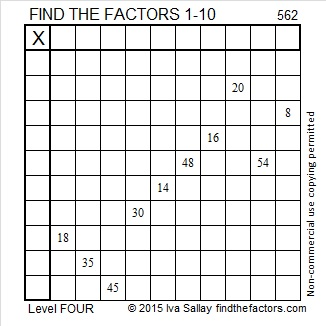Print the puzzles or type the solution on this excel file: 10 Factors 2015-07-20

—————————————————————————————————

• 562 is a composite number.
• Prime factorization: 562 = 2 x 281
• The exponents in the prime factorization are 1 and 1. Adding one to each and multiplying we get (1 + 1)(1 + 1) = 2 x 2 = 4. Therefore 562 has exactly 4 factors.
• Factors of 562: 1, 2, 281, 562
• Factor pairs: 562 = 1 x 562 or 2 x 281
• 562 has no square factors that allow its square root to be simplified. √562 ≈ 23.7065—————————————————————————————————